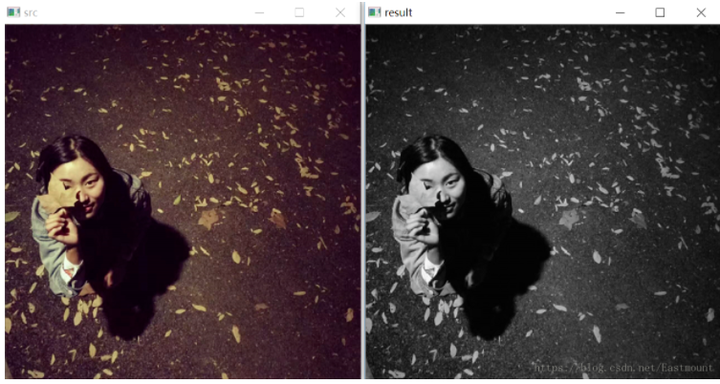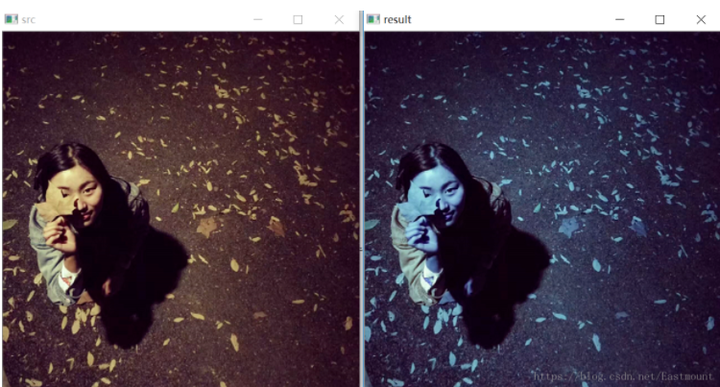# 如何使用Python實現影象融合及加法運算？

## 一.影象加法運算

### 1.Numpy庫加法

1. 當畫素值<=255時，結果為“影象1+影象2”，例如：120+48=168
2. 當畫素值>255時，結果為對255取模的結果，例如：(255+64)%255=64

### 2.OpenCV加法運算

1. 當畫素值<=255時，結果為“影象1+影象2”，例如：120+48=168
2. 當畫素值>255時，結果為255，例如：(255+64) = 255

``````#encoding:utf-8
import cv2
import numpy as np
import matplotlib.pyplot as plt

#讀取圖片
test = img

#方法一：Numpy加法運算
result1 = img + test

#方法二：OpenCV加法運算

#顯示影象
cv2.imshow("original", img)
cv2.imshow("result1", result1)
cv2.imshow("result2", result2)

#等待顯示
cv2.waitKey(0)
cv2.destroyAllWindows()``````## 二.影象融合1. 影象加法：目標影象 = 影象1 + 影象2
2. 影象融合：目標影象 = 影象1 * 係數1 + 影象2 * 係數2 + 亮度調節量

dst = cv2.addWeighter(scr1, alpha, src2, beta, gamma)
dst = src1 * alpha + src2 * beta + gamma

``````#encoding:utf-8
import cv2
import numpy as np
import matplotlib.pyplot as plt

#讀取圖片

#影象融合
result = cv2.addWeighted(src1, 1, src2, 1, 0)

#顯示影象
cv2.imshow("src1", src1)
cv2.imshow("src2", src2)
cv2.imshow("result", result)

#等待顯示
cv2.waitKey(0)
cv2.destroyAllWindows()``````result = cv2.addWeighted(src1, 0.6, src2, 0.8, 10)## 三.影象型別轉換

• cv2.COLOR_BGR2GRAY
• cv2.COLOR_BGR2RGB
• cv2.COLOR_GRAY2BGR

``````#encoding:utf-8
import cv2
import numpy as np
import matplotlib.pyplot as plt

#讀取圖片

#影象型別轉換
result = cv2.cvtColor(src, cv2.COLOR_BGR2GRAY)

#顯示影象
cv2.imshow("src", src)
cv2.imshow("result", result)

#等待顯示
cv2.waitKey(0)
cv2.destroyAllWindows()``````result = cv2.cvtColor(src, cv2.COLOR_BGR2RGB)